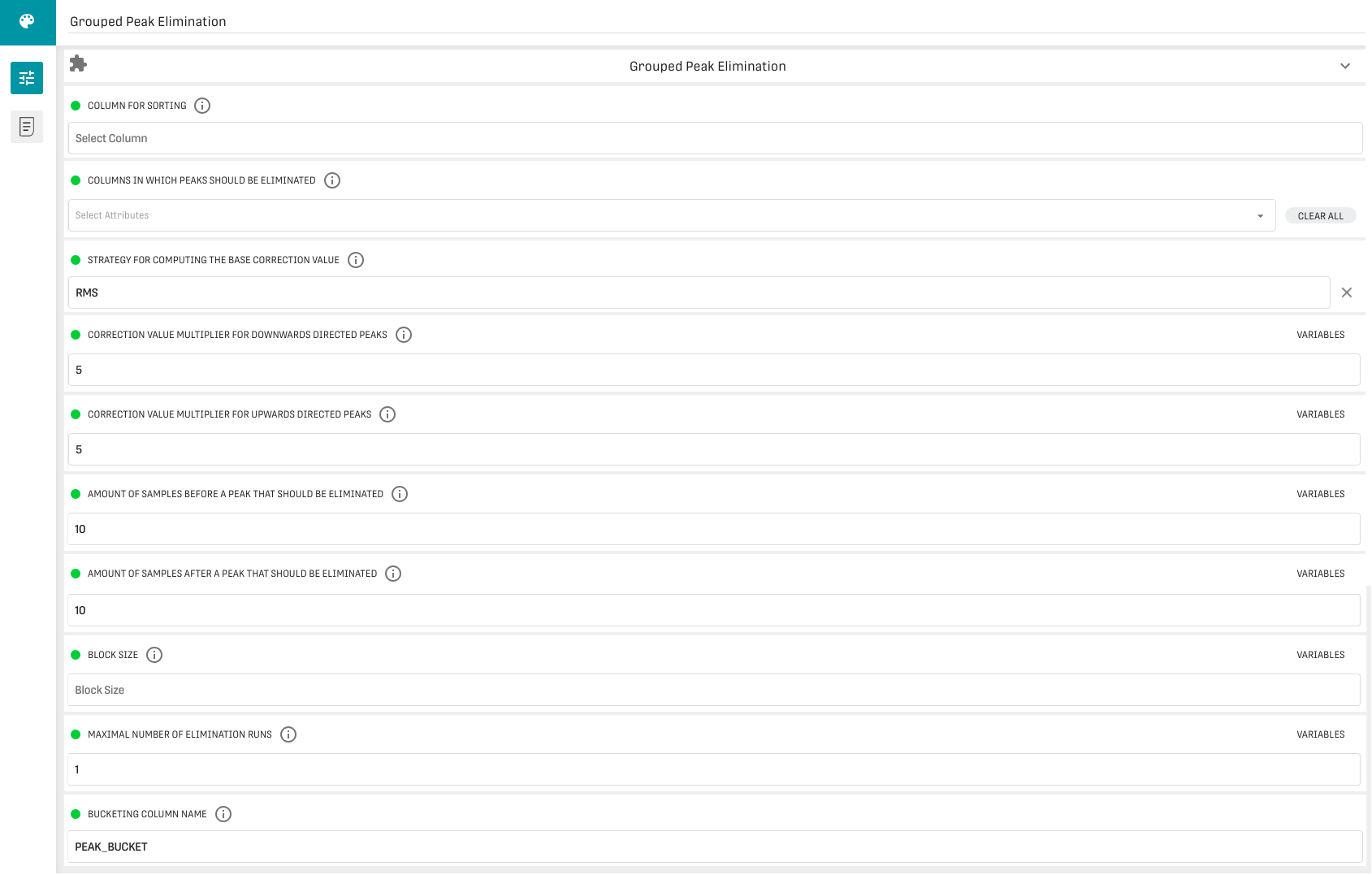# Overview

The Grouped Peak Elimination Processor corrects peaks in the dataset by evaluating the deviation of observations from a calculated correction value. If the deviation is too large, a peak is identified and replaced by interpolated values.

# Input

The processor requires at least one column with numeric values.

# Configuration• Column for Sorting: Choose a column to be used for sorting the data. If no column is set, the original sorting is applied/sorting is not changed.
• Columns in which peaks should be eliminated: Choose the columns in which peaks should be eliminated. If no columns are selected, all columns with a valid type (all numeric columns) are corrected.
• Strategy for computing the base correction value: Choose between MEAN or RMS.
• Correction Value Multiplier for downwards directed peaks: Specify a number which will be multiplied with the computed correction as a threshold for downwards peaks. The value resulting from the multiplication serves as a threshold for the correction. If the distance between the actual value and the normal correction value is larger than the correction value multiplied by this number we have found a downwards directed peak to be corrected.
• Correction Value Multiplier for upwards directed peaks: Specify a number which will be multiplied with the computed correction value as a threshold for upwards peaks.
• Amount of samples before a peak that should be eliminated: Specify a window preceding a peak that will be replaced by the computed correction value.
• Amount of samples after a peak that should be eliminated: Specify a window following a peak that will be replaced by the computed correction value.
• Block Size: Specify a block size used for calculating the correction value. Smaller blocks mean that the correction value will be computed from less samples and vice-versa. If not configured, the window size is chosen by the processor according to the size of the dataset.
• Maximal Number of Elimination Runs: Specify the maximal amount of elimination runs done in one loop. Either if no more peaks can be eliminated or the amount of runs given with this number is reached, the peak elimination stops.
• Bucketing Column Name: Specify a name for an additional column in the output dataset containing numeric values that stand for the buckets in which a given sample was put.

# Output

The processor delivers two output tables: The left output table forwards the original dataset with the bucketing column attached (the name of this column is specified in the last configuration field). The right output table returns information on how many values have been corrected in which window.

Furthermore, the processor itself delivers a table containing information on how many values have been corrected and their percentage w.r.t. overall values.